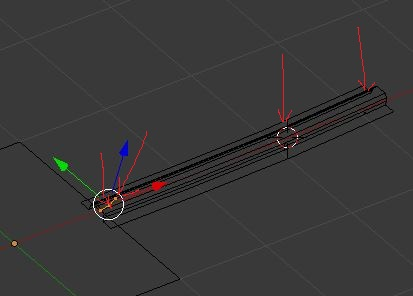# Bmesh translate !

found something here

is there somehow a way to make relative or absolute ?

%%%

I did a loop to extrude and translate some verts
and it is doing it but the location are not good!

``````bmesh.ops.translate(         # Translate
bm,
verts=verts_extrude_b1,
vec=(XC2, YC2, 0))
``````

when using this translate the verts are not where they should be but somewhere else further awayalmost like the values XC2 was multiplied by some value!

any reason for that and how to correct that ?

I can upload the whole class for this I needed
let me know

happy bl

translate is relative.

if you want to move a certain vertex to a certain location, you’ll have to calculate the required relative translation.

now for absolute move there is the
bmesh.ops.transform(bm,matrix, verts)

how do u set up the matrix for a move ?

hope it can be done with that one

may be like that

matrix=Matrix.Translation(1,1,1))
bmesh.ops.transform(bm,matrix=matrix,bm.verts[:])

will the verts include all the other things like edge and faces?

got wrong command
need that one

bmesh.ops.transform(bm, matrix = matrix , space, verts_extrude_b1 )

what do u use for space var ?

thanks

A matrix transformation is just as relative as a translation.

http://www.blender.org/documentation/blender_python_api_2_71_release/bmesh.ops.html#bmesh.ops.transform

you can use a space-matrix to transform in that space, which should usually be the same as multiplying the “matrix”-matrix by the “space”-matrix… seems like a convenience feature of bmesh.ops.transform() to me.

already look at that page and still not following !
any simple example for that
dont’ know what to use for this object matrix thing and what it represents

I also search all scripts on my pc and no example for this new transform bmesh!

thanks

can any one give me an example for this new transform command in latest API
with the space matrix

thanks